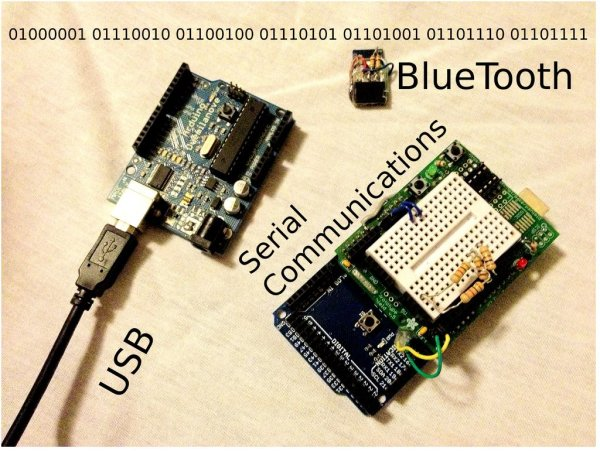# Serial Communications with Arduino

At this point you should have a robot that can think on its own, we need to be able to tap into its thought process. See what he sees. Make some of his decisions for him. in essence, we need to be able to guage his intelligence to make him more intelligent and increase his efficiency.
With serial communications we can do two things: We can either send commands or receive data. Here are some examples to
use with Serial communications:

1. Find out what values your sensors are outputting.
2. Find out if your robot is making the right decisions (Drugs = FALSE;)
3. Change the value of a variable.## Step 1: A BIT of information.

Serial communication works on 1s and 0s. Also known as binary, the Arduino sends these 1s and 0s (bits) one by one, or Serially. These bits are sent in the form of Highs(1) and Lows(0). These bits form together and turn into bytes. A byte is composed of 8 bits. Here’s a little tutorial on binary:
Each bit represents a certain number to add. the first bit (Far right) represents the ones place, similar to the common decimal system.
1 = 1 and 0 = 0.
the next bit’s value is multiplied by two, so:
10 = 2, 11 = 3, 00 = 0 and 01 = 1.

similarly:
100 = 4,
1000 = 8,
10000 = 16,
100000 = 32,
1000000 = 64
10000000 = 128.

Now these bits can form a value up to 255 (11111111).

This value can be turned into ASCII encoded symbols and letters. Here’s a list of them.

READ  Arduino Controlled Bell Tower or Carillon

## Step 2: Serial Output Code

```void setup (){
Serial.begin(9600);
}

void loop(){
Serial.print("The analog value is: ");
delay(100);
}```

Serial Print is a function that prints whatever you specify on the Serial Monitor. In this code, we are going to print the analog value of whatever is in Analog Pin 0.

In the void setup, we begin the serial monitor at a baud rate of 9600.

In the void loop, we are using the Serial.println function and what we are printing is the value found in A0 by the Analog Read.

### Major Components in Project

Arduino

For more detail: Serial Communications with Arduino

#### This Post / Project can also be found using search terms:

• yhs-looksafe_ds_trans